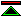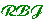# Proofs and Refutations

## The Logic of Mathematical Discovery

### by Imre Lakatos

The purpose of this book is:

to approach some problems of the methodology of mathematics.
where
methodology qua logic of discovery
is intended.

The work, though primarily philosophical in intent, appears also to be in part:

historical
giving logically reconstructed case studies in the development of mathematical theories, with "real history" in the footnotes.
sociological
studying the behaviour of mathematicians and partially classifying the recurrent features in their practice of mathematics
educational analysis and polemic
considering the impact of the presentation of mathematical developments on the comprehension by students of the processes involved, arguing the merits of presentations which retain more of the original structure of the discoveries.
mathematical methodology
arguing that mathematicians practice heuristic rather than deductive methods
mathematical philosophy
arguing against formalism and "dogmatism".
In relation to this objective the material is illuminating, consisting primarily of case studies showing how a putative mathematical discovery can evolve through a series of conjectures, proofs, refutations and reformulations. In this aspect the work has more the character of sociology than philosophy.

The case studies are interpreted through the introduction of special terminology describing recurrent features in the examples cited:

• local and global counterexamples
• monster-barring
• exception-barring
• piecemeal exclusions
• strategic withdrawal
• lemma-incorporation
• proof-generated theorem
• concept-stretching
Alongside and interweaved in this perspective on mathematical discovery we also discover doctrines of a more philosophical nature.

In particular:

the core of this case-study will challenge mathematical formalism

Its modest aim is to elaborate the point that informal, quasi-empirical mathematics does not grow through a monotonous increase of the number of indubitably established theorems, but through the incessant improvement of guesses by speculation and criticism, by the logic of proof and refutation.

It is clear also that Lakatos is attacking:

• Formalism
• dogmatist philosophies of mathematics
• meta-mathematics
• the deductivist approach©created 1995/3/9 modified 1997/2/3